# Telephone Wiretapping

Imagine you are looking to intercept a communication that can happen between two people over a telephone network. Let’s say that the two people in question are part of a larger group, who all communicate with each other (sometimes via other people in the network if they don’t have their phone number). We can represent this as a graph where the vertices are people and edges connect two people if they have each other in their phone books.

Here’s a network with `6` people, some of whom don’t directly communicate with each other but can do so through others. Each person can reach all the others though, so the graph is connected.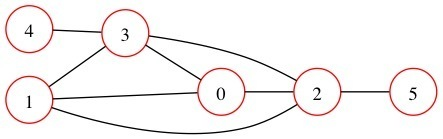Let’s also assume that this group of people communicate efficiently and use the smallest amount of calls possible and always distribute information to every person. If an unknown member of the network wants to communicate some nefarious plans to all the other members they call some people who in turn spread the message through the network by making more calls while adhering to the rules above. If we can tap a single link between two people what is the probability of intercepting one of these calls? Let’s work through an example of a network of 4 people who can each communicate with two others. The graph looks like this: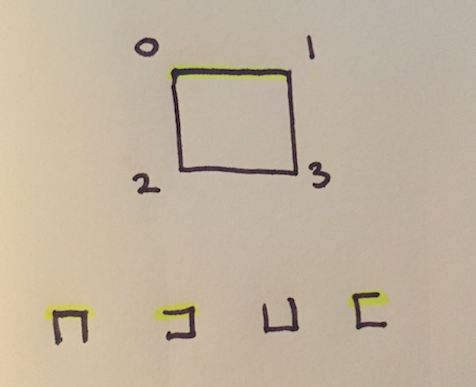If we tap then link connecting `0` and `1` there is only one way to communicate to all members without using this tapped link, where as there are `3` that do use it. Meaning that the probability of intercepting the information is `0.75`. The small images represent the ways in which the communication can happen and those that use the tapped link (will be intercepted) are highlighted.

There are a couple of important things to note as this point. Firstly the links chosen to communicate over form a spanning tree of the graph. This is an important property as a spanning tree has one less edge than the number of nodes and doesn’t contain any cycles. Cycles would mean that the communication has not been efficient because we could remove an edge on the cycle and still have the information reach all the people.

Let’s work through another example and compute the probability of intercepting the communication if we tap a specific link. Here is another graph. It represents `4` people but this time there are `6` links. Everyone can communicate with everyone else. Let’s tap the top link - highlighted in yellow.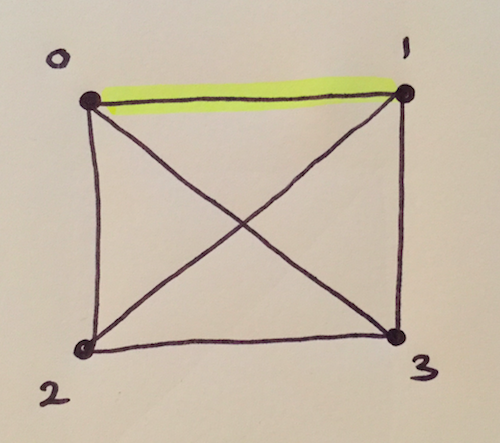Now let’s enumerate all the spanning trees of this graph manually. Notice that each spanning tree connects all the vertices in the original graph just using fewer edges. In particular `3` edges which is one less that the number of vertices. Adding another edge would create a cycle. There are `16` different spanning trees and `8` of them (highlighted in yellow) use the link we have tapped. This means the probability of intercepting the transitions is `8.0 / 16.0 = 0.5`.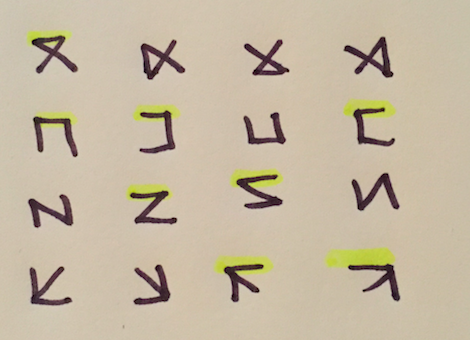Cool! So to solve this problem we need to count the number of spanning trees of a graph that uses a specified edge - call that value `A`. Then compute the number of spanning trees that the graph has - call that value `B`. The probability of intercepting the communication on the tapped link is `A/B`.

The number of spanning trees that use a specific edges can be computed by collapsing the vertices at each end of that edge into one vertex and computing the number of spanning trees for that new multi-graph. For example for the cross-box graph above if we want to find the number of spanning trees that use the top edge we collapse it and generate the graph on the right which indeed has `8` spanning trees. Remember this could create multi edges between vertices.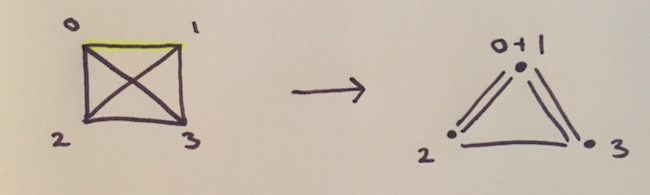Enumerating all the spanning trees is not a feasible option as this number grows really quickly. In fact Cayley’s formula gives the number of spanning trees of a complete graph of size `K` as `K ** (K-2)`.

Instead we can use Kirchhoff’s matrix tree theorem. Which tells us that if we have a graph represented by an adjacency matrix `G` we can count the number of the spanning trees as:Where the lambdas are the non-zero eigenvalues of the associated Laplacian matrix `G`. It’s actually easier and more numerically stable to compute the determinant of a cofactor of the Laplacian which gives the same result. The Laplacian matrix is used to compute lots of useful properties of graphs. It is equal to the degree matrix minus the adjacency matrix:Computing the Laplacian from an adjacency matrix can be done with this code:

``````# compute the Laplacian of the adjacency matrix
def laplacian(A):
L = -A

for a in xrange(L.shape):
for b in xrange(L.shape):
if A[a][b]:
L[a][a] += A[a][b] # increase degree

return L
``````

Using this we can compute the cofactor.

``````def cofactor(L, factor=1.0):
Q = L[1::, 1::] # bottom right minor
return np.linalg.det(Q / factor)
``````

Also I added a scaling parameter to the cofactor computation. The determinants can get really big when the network has thousands of vertices. In this case computing the numerator and denominator of the probability can result in overflow. If we take some factor `factor` out of the Laplacian matrices before computing the determinant we reduce the value by `factor ** N` where N is the size of the matrix. Using this we can compute the probability for large matrices because the factors almost totally cancel out because the matrices dimensions different by only 1.

``````def probability(G1, G2):
factor = 24.0

# det(A) = f**n*det(A/f)
L1 = laplacian(G1)
L2 = laplacian(G2)
Q1 = cofactor(L1, factor=factor)
Q2 = cofactor(L2, factor=factor)

# f**(n-1) * det(G1/f)
# -------------------
# f**(n-2) * det(G2/f)
return Q1 / Q2 / factor
``````

Using this we can go through each edge in the graph and compute the probability of intercepting if we tap that edge. This value will change depending on the graph and if it has an articulating points these edges will have probability `1.0`.